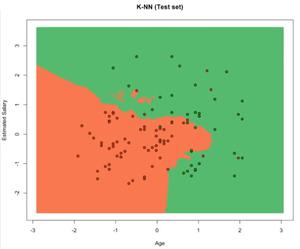GeeksforGeeks App
Open AppBrowser
Continue

# Regression using k-Nearest Neighbors in R Programming

Machine learning is a subset of Artificial Intelligence that provides a machine with the ability to learn automatically without being explicitly programmed. The machine in such cases improves from the experience without human intervention and adjusts actions accordingly. It is primarily of 3 types:

#### K-Nearest Neighbors

The K-nearest neighbor algorithm creates an imaginary boundary to classify the data. When new data points are added for prediction, the algorithm adds that point to the nearest of the boundary line. It follows the principle of “Birds of a feather flock together.” This algorithm can easily be implemented in the R language.

#### K-NN Algorithm

1. Select K, the number of neighbors.
2. Calculate the Euclidean distance of the K number of neighbors.
3. Take the K nearest neighbors as per the calculated Euclidean distance.
4. Count the number of data points in each category among these K neighbors.
5. The new data point is assigned to the category for which the number of the neighbor is maximum.

### Implementation in R

The Dataset: A sample population of 400 people shared their age, gender, and salary with a product company, and if they bought the product or not(0 means no, 1 means yes). Download the dataset Advertisement.csv

## R

 `# Importing the dataset``dataset = ``read.csv``(``'Advertisement.csv'``)``head``(dataset, 10)`

Output:

## R

 `# Encoding the target``# feature as factor``dataset\$Purchased = ``factor``(dataset\$Purchased,``                           ``levels = ``c``(0, 1))`` ` `# Splitting the dataset into ``# the Training set and Test set``# install.packages('caTools')``library``(caTools)``set.seed``(123)``split = ``sample.split``(dataset\$Purchased, ``                     ``SplitRatio = 0.75)``training_set = ``subset``(dataset, ``                      ``split == ``TRUE``)``test_set = ``subset``(dataset, ``                  ``split == ``FALSE``)`` ` `# Feature Scaling``training_set[-3] = ``scale``(training_set[-3])``test_set[-3] = ``scale``(test_set[-3])`` ` `# Fitting K-NN to the Training set ``# and Predicting the Test set results``library``(class)``y_pred = ``knn``(train = training_set[, -3],``             ``test = test_set[, -3],``             ``cl = training_set[, 3],``             ``k = 5,``             ``prob = ``TRUE``)`` ` `# Making the Confusion Matrix``cm = ``table``(test_set[, 3], y_pred)`

• The training set contains 300 entries.
• The test set contains 100 entries.
```Confusion matrix result:
[
]

```

Visualizing the Training Data:

## R

 `# Visualising the Training set results``# Install ElemStatLearn if not present ``# in the packages using(without hashtag)``# install.packages('ElemStatLearn')``library``(ElemStatLearn)``set = training_set`` ` `#Building a grid of Age Column(X1)``# and Estimated Salary(X2) Column``X1 = ``seq``(``min``(set[, 1]) - 1,``         ``max``(set[, 1]) + 1,``         ``by = 0.01)``X2 = ``seq``(``min``(set[, 2]) - 1, ``         ``max``(set[, 2]) + 1, ``         ``by = 0.01)``grid_set = ``expand.grid``(X1, X2)`` ` `# Give name to the columns of matrix``colnames``(grid_set) = ``c``(``'Age'``,``                       ``'EstimatedSalary'``)`` ` `# Predicting the values and plotting``# them to grid and labelling the axes``y_grid = ``knn``(train = training_set[, -3],``             ``test = grid_set,``             ``cl = training_set[, 3],``             ``k = 5)``plot``(set[, -3],``     ``main = ``'K-NN (Training set)'``,``     ``xlab = ``'Age'``, ylab = ``'Estimated Salary'``,``     ``xlim = ``range``(X1), ylim = ``range``(X2))``contour``(X1, X2, ``matrix``(``as.numeric``(y_grid), ``                       ``length``(X1), ``length``(X2)),``                       ``add = ``TRUE``)``points``(grid_set, pch = ``'.'``,``       ``col = ``ifelse``(y_grid == 1, ``                    ``'springgreen3'``, ``'tomato'``))``points``(set, pch = 21, bg = ``ifelse``(set[, 3] == 1, ``                                  ``'green4'``, ``'red3'``))`

Output:Visualizing the Test Data:

## R

 `# Visualising the Test set results``library``(ElemStatLearn)``set = test_set`` ` `# Building a grid of Age Column(X1)``# and Estimated Salary(X2) Column``X1 = ``seq``(``min``(set[, 1]) - 1,``         ``max``(set[, 1]) + 1, ``         ``by = 0.01)``X2 = ``seq``(``min``(set[, 2]) - 1,``         ``max``(set[, 2]) + 1, ``         ``by = 0.01)``grid_set = ``expand.grid``(X1, X2)`` ` `# Give name to the columns of matrix``colnames``(grid_set) = ``c``(``'Age'``, ``                       ``'EstimatedSalary'``)`` ` `# Predicting the values and plotting ``# them to grid and labelling the axes``y_grid = ``knn``(train = training_set[, -3], ``             ``test = grid_set,``             ``cl = training_set[, 3], k = 5)``plot``(set[, -3],``     ``main = ``'K-NN (Test set)'``,``     ``xlab = ``'Age'``, ylab = ``'Estimated Salary'``,``     ``xlim = ``range``(X1), ylim = ``range``(X2))``contour``(X1, X2, ``matrix``(``as.numeric``(y_grid), ``                       ``length``(X1), ``length``(X2)),``                       ``add = ``TRUE``)``points``(grid_set, pch = ``'.'``, col = ``       ``ifelse``(y_grid == 1, ``              ``'springgreen3'``, ``'tomato'``))``points``(set, pch = 21, bg =``       ``ifelse``(set[, 3] == 1,``              ``'green4'``, ``'red3'``))`

Output:1. There is no training period.
• KNN is an instance-based learning algorithm, hence a lazy learner.
• KNN does not derive any discriminative function from the training table, also there is no training period.
• KNN stores the training dataset and uses it to make real-time predictions.
2. New data can be added seamlessly and it will not impact the accuracy of the algorithm as there is no training needed for the newly added data.
3. There are only two parameters required to implement the KNN algorithm i.e. the value of K and the Euclidean distance function.